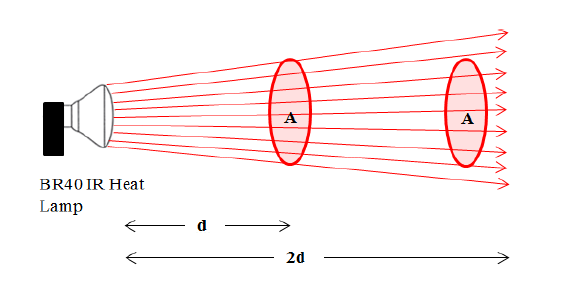## Heat Increasing Rate and Distance

In this post, we talk about Heat Increasing Rate and Distance.

The temperature in the distance of 1.5m was growing three times faster than the temperature measured in the distance of 3.0 m. The phenomenon happens because the rate of light concentration for a fixed area and the rate of energy loss depend on the distance between the heat source and the object. The heat lamp is a common reflector type bulb (Bulged Reflector (BR) series, one of the standard light bulb shape) shape which lets the light disperse more for the further distance so that the heat concentration gets lower when the object moves away. Consequently, the energy loss increases even if the IR heat lamp generates radiant heat. Figure 58 shows the heat concentration for the same size of the area in the different distances. ‘A’ refers the area of the ray contacting surface and the variable, d, is the value of the distance between the heat core and the object. As shown in this simple figure, the heat amount decreases.To reduce the energy loss by dispersing the rays, limited the heat spreading area by using the focusing cap. The heat lamp’s output rays were focused when the case bounds the rays’ spreading angle and  you can adjust the focused area by considering length and shape of the cap and the light reflecting rate and direction of inner frame.